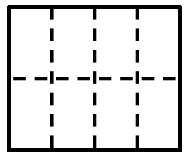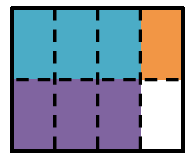### Home > CC2MN > Chapter 2 > Lesson 2.2.5 > Problem2-90

2-90.

Frieda Friendly works for a local car dealership. She noticed that $\frac { 3 } { 4 }$ of the cars are sedans and that half of them are white. What fraction of the dealership’s cars are white sedans?

1. Use a unit rectangle to represent this situation. Label the parts carefully.

Draw a rectangle. A rectangle is drawn to the left.

Subdivide the rectangle and label appropriately. The rectangle is divided into 2 rows and 4 columns of 8 equal parts

One dimension will represent the dealership's different types of cars, while the other will represent the dealership's different colors of cars.

Shade half of them to represent the white cars, and three-fourths to represent the sedans. The area of the overlapping region is the answer. Shading added as follows: 3 left columns of top row, blue, last column of top row, orange, 3 left columns of bottom row, purple.2. Write an equation or a sentence that describes the situation, and answer the question.

Write an equation that finds the area of the overlapping region. Then you can find the answer.
See problem 2-89 for examples.

$\frac{3}{4}\cdot\frac{1}{2}=\frac{3}{8}$

3. Write your answer from part (b) as a decimal and as a percent.

Convert the fraction into a decimal by dividing the numerator by the denominator. Convert the decimal into a percent by multiplying it by $100\%$ or moving the decimal.